# Relational Data Modeling - One-to-One Relationship

A one-to-one relationship is a relational relationship that is equivalent to the set bijection relationship

This relationship describe that one row from a relation point to exactly one row to the other relation.

## Modeling

There are severals way to model a one-to-one relationship:

As a relation itself is a one-to-one relational structure, it means that the two relation (tables) could also be merge in one.

Recommended Pages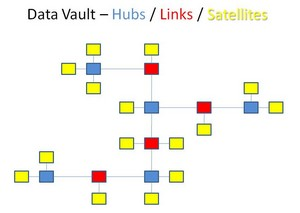Data Vault - Satellite

Satellite are tables in the data vault: that hold all the descriptive attribute and their history in a scd2 form A satellite can be created from all descriptive attributes of a table source such...Dimensional Data Modeling - Degenerate Dimension of Fact dimension (i.e. event/header entity)

In a dimensional model, you may find a dimension table: with a cardinality (distinct value is higher than 10,000). cardinality which has a many-to-one relationship with the fact table close to a one-to-one...Dimensional Data Modeling - Relationship

In a dimensional data model, you have mostly a one-to-many relationship between the dimension and the fact table between each level in a hierarchy man-to-many relationship are modeled with a bridge...Index - Btree structure (Balanced Tree)

BTree indexes : has a tree structure provides fast access, by key, to an individual row or range of rows normally requiring few reads to find the correct row are similar in implementation to a...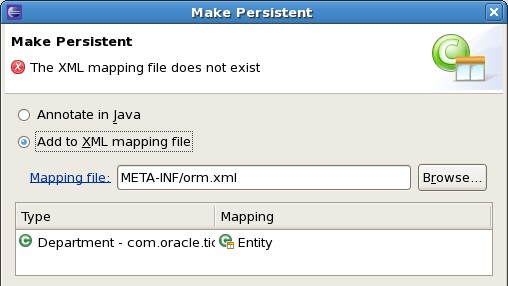JPA - Entity Annotations

A key feature of EJB 3.0 and JPA is the ability to create entities that contain object-relational mappings by using (metadata) annotations rather than deployment descriptors (orm.xml) as in earlier versions....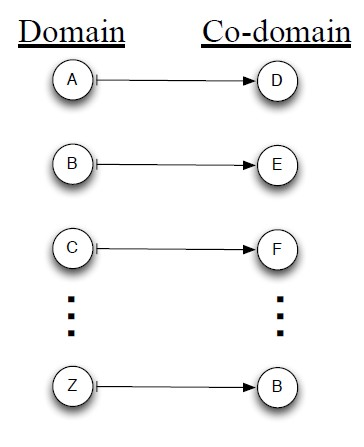Linear Algebra - Function (Set)

For each input element in a set , a function assigns a single output element from another set . is called the domain of the function is called the co-domain or in Mathese: The function...Ordinal Data - Order type

In mathematics, especially in set theory, two ordered sets X,Y are said to have the same order type just when they are order isomorphic, that is, when there exists a bijection f: X → Y such that both...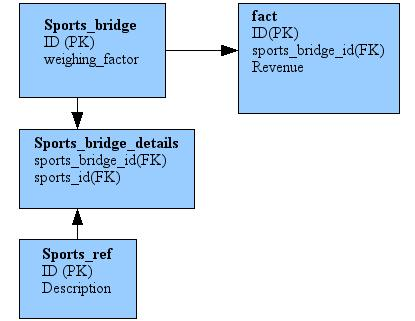Relational Data Modeling - Association Table (Bridge, Cross)

An association table (bridge table) is a table that permits to implement: one-to-one relationship or many-to-many relationship Because most of database only support one-to-many relationships, it...Relational Data Modeling - Cardinality

The cardinality is way to define the relationship between two relation in a data model : one-to-one optional on one side one-to-one one-to-many many-to-many ... Cardinalities further describe...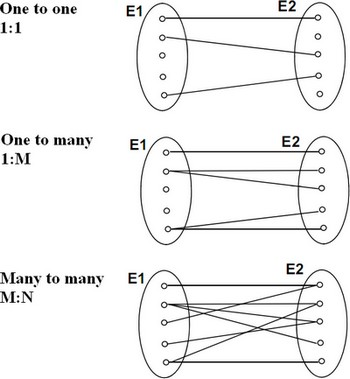Relational Data Modeling - Relationship

relationship in a relational model. relation (generally a table) an entity or a relationship cardinality define the type of relationships between two relations. third junction table (association...